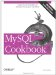# Generating Frequency Distributions

13.4.1 Problem

You want to know the frequency of occurrence for each value in a table.

13.4.2 Solution

Derive a frequency distribution that summarizes the contents of your dataset.

13.4.3 Discussion

A common application for per-group summary techniques is to generate a breakdown of the number of times each value occurs. This is called a frequency distribution. For the testscore table, the frequency distribution looks like this:

```mysql> SELECT score, COUNT(score) AS occurrence
-> FROM testscore GROUP BY score;
+-------+------------+
| score | occurrence |
+-------+------------+
| 4 | 2 |
| 5 | 1 |
| 6 | 4 |
| 7 | 4 |
| 8 | 2 |
| 9 | 5 |
| 10 | 2 |
+-------+------------+```

If you express the results in terms of percentages rather than as counts, you produce a relative frequency distribution. To break down a set of observations and show each count as a percentage of the total, use one query to get the total number of observations, and another to calculate the percentages for each group:

```mysql> SELECT @n := COUNT(score) FROM testscore;
mysql> SELECT score, (COUNT(score)*100)/@n AS percent
-> FROM testscore GROUP BY score;
+-------+---------+
| score | percent |
+-------+---------+
| 4 | 10 |
| 5 | 5 |
| 6 | 20 |
| 7 | 20 |
| 8 | 10 |
| 9 | 25 |
| 10 | 10 |
+-------+---------+```

The distributions just shown summarize the number of values for individual scores. However, if the dataset contains a large number of distinct values and you want a distribution that shows only a small number of categories, you may wish to lump values into categories and produce a count for each category. "Lumping" techniques are discussed in Recipe 7.13.

One typical use of frequency distributions is to export the results for use in a graphing program. In the absence of such a program, you can use MySQL itself to generate a simple ASCII chart as a visual representation of the distribution. For example, to display an ASCII bar chart of the test score counts, convert the counts to strings of * characters:

```mysql> SELECT score, REPEAT('*',COUNT(score)) AS occurrences
-> FROM testscore GROUP BY score;
+-------+-------------+
| score | occurrences |
+-------+-------------+
| 4 | ** |
| 5 | * |
| 6 | **** |
| 7 | **** |
| 8 | ** |
| 9 | ***** |
| 10 | ** |
+-------+-------------+```

To chart the relative frequency distribution instead, use the percentage values:

```mysql> SELECT @n := COUNT(score) FROM testscore;
mysql> SELECT score, REPEAT('*',(COUNT(score)*100)/@n) AS percent
-> FROM testscore GROUP BY score;
+-------+---------------------------+
| score | percent |
+-------+---------------------------+
| 4 | ********** |
| 5 | ***** |
| 6 | ******************** |
| 7 | ******************** |
| 8 | ********** |
| 9 | ************************* |
| 10 | ********** |
+-------+---------------------------+```

The ASCII chart method is fairly crude, obviously, but it's a quick way to get a picture of the distribution of observations, and it requires no other tools.

If you generate a frequency distribution for a range of categories where some of the categories are not represented in your observations, the missing categories will not appear in the output. To force each category to be displayed, use a reference table and a LEFT JOIN (a technique discussed in Recipe 12.10). For the testscore table, the possible scores range from 0 to 10, so a reference table should contain each of those values:

```mysql> CREATE TABLE ref (score INT);
mysql> INSERT INTO ref (score)
-> VALUES(0),(1),(2),(3),(4),(5),(6),(7),(8),(9),(10);```

Then join the reference table to the test scores to generate the frequency distribution:

```mysql> SELECT ref.score, COUNT(testscore.score) AS occurrences
-> FROM ref LEFT JOIN testscore ON ref.score = testscore.score
-> GROUP BY ref.score;
+-------+-------------+
| score | occurrences |
+-------+-------------+
| 0 | 0 |
| 1 | 0 |
| 2 | 0 |
| 3 | 0 |
| 4 | 2 |
| 5 | 1 |
| 6 | 4 |
| 7 | 4 |
| 8 | 2 |
| 9 | 5 |
| 10 | 2 |
+-------+-------------+```

This distribution includes rows for scores 0 through 3, none of which appear in the frequency distribution shown earlier.

The same principle applies to relative frequency distributions:

```mysql> SELECT @n := COUNT(score) FROM testscore;
mysql> SELECT ref.score, (COUNT(testscore.score)*100)/@n AS percent
-> FROM ref LEFT JOIN testscore ON ref.score = testscore.score
-> GROUP BY ref.score;
+-------+---------+
| score | percent |
+-------+---------+
| 0 | 0 |
| 1 | 0 |
| 2 | 0 |
| 3 | 0 |
| 4 | 10 |
| 5 | 5 |
| 6 | 20 |
| 7 | 20 |
| 8 | 10 |
| 9 | 25 |
| 10 | 10 |
+-------+---------+```MySQL Cookbook
ISBN: 059652708X
EAN: 2147483647
Year: 2005
Pages: 412
Authors: Paul DuBois